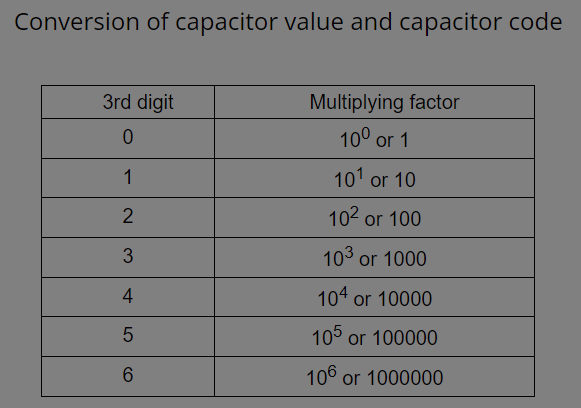(206) 687-4009 | [email protected]

# CAPACITOR VALUE & COLOR CODE CALCULATOR

Ceramic calculators are usually small in size. So it is not possible to write their value on the capacitor. So they are coded using 3 digits code. These codes can be encoded to determine their value using the capacitor code to value calculator.

Choose what to calculate

Enter 3 digit capacitor codeChoose what to calculate

Capacitance (C)## Capacitor value and code calculator parameter:

• Choose what to calculate:CAPACITOR VALUE or CAPACITOR CODE
• If capacitor value needs to be calculated:enter 3 digit capacitor codes and result appears in Pico farad, Nano farad and micro farad.
• If capacitor code needs to be calculated:enter capacitance value in either Pico, Nano or micro farad and result is 3 digit capacitor code.

## Conversion of capacitor value and capacitor code:

Following steps help in converting capacitor value to capacitor code or vice versa:

• The value of the capacitor in Pico farad is the first two-digit of code
• The 3rd digit is the multiplying factor ranging from 0 to 6.
3rd digit Multiplying factor
0 100 or 1
1 101 or 10
2 102 or 100
3 103 or 1000
4 104 or 10000
5 105 or 100000
6 106 or 1000000
• The above coding is valid only when capacitor value is provided in Pico farad. If values are provided in micro or Nano farad then it is converted into Pico farad and then coding is done.

## Solved Example:

### For capacitor value to capacitor code conversion:

1. When capacitor value is given in Pico farad:

Given:

Capacitor value = 12 pf

Required:

Capacitor code=?

Solution:

Capacitor code=120

2.When capacitor value is given in Nano farad:

Given:

Capacitor value = 47 nF

Required:

Capacitor code=?

Solution:

Capacitor value =47*10^3 pF (Nano to Pico conversion)

Capacitor code=473

3.When capacitor value is given in Nano farad:

Given:

Capacitor value = 3.3 µF

Required:

Capacitor code=?

Solution:

Capacitor value =3.3*10^6 pF (micro to Pico conversion)

Capacitor value=3300000 or 33*10^5

Capacitor code=335

### For capacitor code to capacitor value conversion:

Given:

Capacitor code=234

Required:

Capacitor code=?

Solution:

Capacitor value=23*10^4 pF

Capacitor value =
23*104 / 103
or 230 nF (1pico =
1 / 103
nano)
Capacitor value =
23*104 / 106
or 0.23 µF (1pico =
1 / 106
micro)